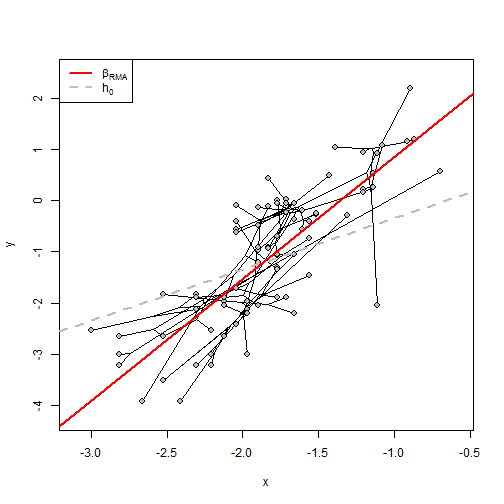## Tuesday, April 11, 2017

### Fix to `plot` method for `"phyl.RMA"` object class

I just fixed a small bug in the S3 `plot` method for `phyl.RMA`.

`phyl.RMA` does reduced major axis (RMA) regression taking phylogeny into account. The `plot` method plots a phylomorphospace of the two characters, and overlays both the null hypothesis (to be specified by the user, but defaults to β1 = 1.0) and the fitted RMA regression line.

Here's in example starting with a fitted RMA model:

``````library(phytools)
obj
``````
``````##
## Coefficients:
## (Intercept)           x
##    3.214059    2.375175
##
## VCV matrix:
##             x           y
## x 0.005793694 0.006437997
## y 0.006437997 0.032684872
##
## Model for the covariance structure of the error is "BM"
##
## Estimates (or set values):
##    lambda    log(L)
##    1.0000 -107.8858
##
## Hypothesis test based on Clarke (1980; Biometrika):
##        r2         T        df         P
##  0.218877  8.754613 74.108564  0.000000
##
## Note that the null hypothesis test is h0 = 1
``````
``````plot(obj)
``````The fix guarantees that both the null hypothesis & fitted model RMA regression lines pass through the ML reconstructed ancestral state value for the root node of both x & y. (For some reason, this was not the case before - although it should have been.)